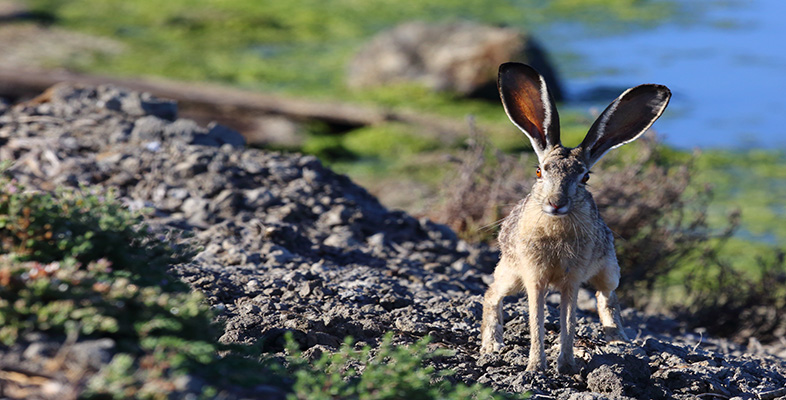Hearing

Start this free course now. Just create an account and sign in. Enrol and complete the course for a free statement of participation or digital badge if available.

Free course

# Hearing

The procedure for a masking experiment is shown in Figure 38. First, the threshold for hearing is determined across a range of frequencies (Figure 38a). Then, a masking stimulus is presented at a particular place along the frequency scale and while the masking stimulus is sounding, the thresholds for all frequencies are re-determined (Figure 38b). The masking stimulus used can be a pure tone or, more commonly white noise. A white noise stimulus is simply one that contains a band of frequencies with equal sound pressure at each frequency. It sounds something like the ‘shhhhhhh’ sound you can make by blowing air across your teeth when they are slightly separated. The band of frequencies used can vary in width. For example, a band of frequencies 90 Hz wide centred on 410 Hz would contain frequencies ranging from 365 to 455 Hz.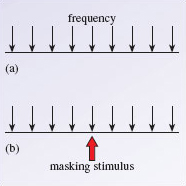Figure 38 The procedure for a masking experiment. (a) The threshold is determined across a range of frequencies. Each arrow indicates a frequency where the threshold is measured. (b) The threshold is re-determined at each frequency (small arrows) in the presence of a masking stimulus (large arrow)

When thresholds are measured in the presence of a masking noise the original thresholds are raised. Figure 39 shows the result of an experiment using a 90 Hz band of noise centred at 410 Hz.

There are two things to note about Figure 39. First, the threshold increases most for frequencies near the frequencies in the masking tone. Second, the curve is not symmetrical; the masking effect spreads more to high frequencies than to low frequencies. So, lower frequencies mask higher-frequency sounds much more effectively than the reverse.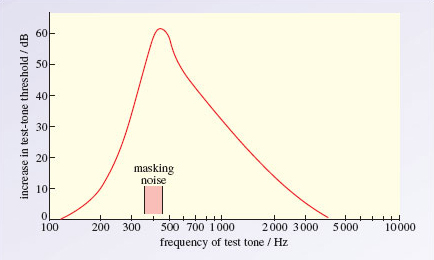Figure 39 Results of a masking experiment. The red line indicates the amount that the threshold is raised in the presence of a masking noise centred at 410 Hz. So for a 410 Hz tone, the threshold is raised by about 60 dB above absolute threshold

How is this masking effect explained?

Many masking effects can be explained in a very simplified way by analysing the interaction of displacement patterns in response to sound on the basilar membrane. Figure 40 shows the vibration patterns on the basilar membrane caused by a 400 Hz, 800 Hz and 1000 Hz tone. You can see that the vibration pattern of the 800 Hz tone overlaps those of the 400 and 1000 Hz tones. Note also that the pattern for the 800 Hz tone (which is shaded) almost totally overlaps the pattern for the higher frequency, 1000 Hz tone, but does not overlap the place of peak vibration of the lower frequency, 400 Hz tone. We would therefore expect the 800 Hz tone to mask the 1000 Hz tone more effectively than the 400 Hz tone. This is what happens, providing support for the place mechanism of frequency tuning on the basilar membrane.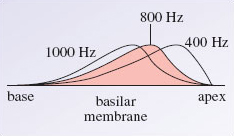Figure 40 Vibration patterns on the basilar membrane caused by 400, 800 and 1000 Hz tones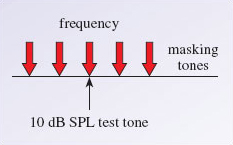Figure 41 The procedure for measuring a psychophysical tuning curve. A10 dB test tone (black arrow) is presented and then a series of masking tones (red arrows) are presented at the same time as the test tone. The psychophysical tuning curve is generated by determining the SPL threshold of the masking tones needed to reduce the perception of the test tone to threshold

The close match between them suggests that both reflect the same process – a place code for frequency on the basilar membrane.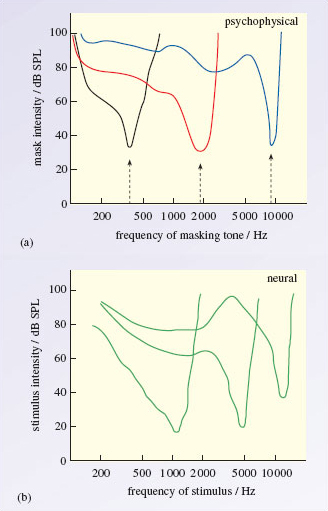Figure 42 (a) Three human psychophysical tuning curves generated using the method described in Figure 41. The arrows show the frequency of three different test tones. You can see from the figure that when the masking tone is the same as, or close to, the test tone in frequency, the intensity of the masker needed to mask the test tone is low. (b) Three neural tuning curves showing the stimulus intensity needed to generate a constant response (firing rate) in the nerve fibre of a cat. Each curve represents a different auditory nerve fibre